# NCERT Solutions for Class 6 Maths Chapter 6 Integers (Ex 6.1) Exercise 6.1

## NCERT Solutions for Class 6 Maths Chapter 6 Integers (Ex 6.1) Exercise 6.1

Free PDF download of NCERT Solutions for Class 6 Maths Chapter 6 Exercise 6.1 (Ex 6.1) and all chapter exercises at one place prepared by an expert teacher as per NCERT (CBSE) books guidelines. Class 6 Maths Chapter 6 Integers Exercise 6.1 Questions with Solutions to help you to revise complete Syllabus and Score More marks. Register and get all exercise solutions in your emails.

Every NCERT Solution is provided to make the study simple and interesting on Vedantu. Subjects like Science, Maths, English,Hindi will become easy to study if you have access to NCERT Solution for Class 6 Science , Maths solutions and solutions of other subjects.

Do you need help with your Homework? Are you preparing for Exams?
Study without Internet (Offline)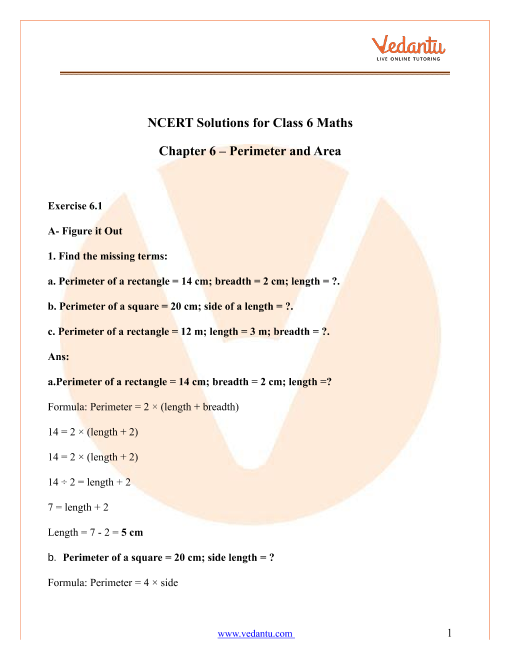Book your Free Demo session
Get a flavour of LIVE classes here at VedantuVedantu Improvement Promise
We promise improvement in marks or get your fees back. T&C Apply*

## NCERT Solutions for Class 6 Maths Chapter 6 Integers Exercise 6.1

Exercise 6.1

1. Write opposite of the following:

(i) Increase in weight

Ans: Opposite of a statement means something that conveys the exact reverse sense.

The given statement is, ‘Increase in weight’. This means that the amount of weight is rising. The opposite of this would be that the amount of weight is reduced. This means that the opposite of increase is decrease. So, the opposite of the given statement will decrease in weight. Therefore, the opposite of the given statement, ‘Increase in weight’ is ‘Decrease in weight’.

(ii) 30 km North

Ans: Opposite of a statement means something that conveys the exact reverse sense.

The given statement is, ’30 km North’. This means that the direction is 30 kms towards the North. The opposite of this would be that the direction is 30 km towards the South. This means that the opposite of North is South. So, the opposite of the given statement will be 30 km South. Therefore, the opposite of the given statement, ‘’30 km North’ is ‘’30 km South’.

(iii) 326 BC

Ans: Opposite of a statement means something that conveys the exact reverse sense.

The given statement is, ’326 BC’. This means 326 years before Jesus Christ was born. The opposite of this would be 326 years after Jesus Christ was born which is known as 326 AD. This means that the opposite of BC is AD. So, the opposite of the given statement will be 326 AD. Therefore, the opposite of the given statement, ‘326 BC’ is ‘326 AD’.

(iv) Loss of Rs. 700

Ans: Opposite of a statement means something that conveys the exact reverse sense.

The given statement is, ‘Loss of Rs. 700’. This means that the amount of Rs. 700 is lost. The opposite of this would be that the amount of Rs. 700 is gained which is known as profit. This means that the opposite of loss is profit. So, the opposite of the given statement will be profit of Rs. 700. Therefore, the opposite of the given statement, ‘Loss of Rs. 700’ is ‘Profit of Rs. 700’.

(v) 100 m above sea level

Ans: Opposite of a statement means something that conveys the exact reverse sense.

The given statement is, ‘100 m above sea level’. This means that the distance is over the sea level by 100 m. The opposite of this would be that the distance is beneath the sea level by 100 m. This means that the opposite of above is below. So, the opposite of the given statement will be 100 m below sea level.

Therefore, the opposite of the given statement, ‘100 m above sea level’ is ‘100 m below sea level’.

2. Represent the following numbers as integers with appropriate signs.

(i) An aeroplane is flying at a height two thousand meters above the ground.

Ans: Integer is a set of both positive and negative numbers including 0.

The given statement is, ‘An aeroplane is flying at a height two thousand meters above the ground’.

The numerical part in the given statement is 2000 m.

We will now assign a sign system to denote the given number with a sign and make it integer.

Let us assume that the height above the ground level is positive and the height below the ground level is negative. Here the ground level is taken as the zero level.

Since the airplane is flying above the ground level at a height of 2000 meters, the distance will be taken as positive.

Therefore, the representation of the number as an integer with the sign is $\left( + \right)2000\,\text{m}$.

(ii) A submarine is moving at a depth eight thousand meters below the sea level.

Ans: Integer is a set of both positive and negative numbers including 0.

The given statement is, ‘A submarine is moving at a depth eight thousand meters below the sea level’.

The numerical part in the given statement is 8000 m.

We will now assign a sign system to denote the given number with a sign and make it integer.

Let us assume that the height above the ground level is positive and the height below the ground level is negative. Here the ground level is taken as the zero level.

Since the submarine is moving below the sea level at a depth of 8000 meters, the distance will be taken as negative.

Therefore, the representation of the number as an integer with the sign is $\left( - \right)8000\,\text{m}$.

(iii) A deposit of rupees two hundred.

Ans: Integer is a set of both positive and negative numbers including 0.

The given statement is, ‘A deposit of rupees two hundred’.

The numerical part in the given statement is Rs. 200.

We will now assign a sign system to denote the given number with a sign and make it integer.

Let us assume that the deposit of rupees is positive and the withdrawal of rupees is negative. Here the current balance will be considered as zero and any transaction will be made against it.

Since Rs. 200 is deposited, the transfer will be taken as positive.

Therefore, the representation of the number as an integer with the sign is $\left( + \right)200\,\text{Rs}\text{.}$

(iv) Withdrawal of rupees seven hundred.

Ans: Integer is a set of both positive and negative numbers including 0.

The given statement is, ‘Withdrawal of rupees seven hundred’.

The numerical part in the given statement is Rs. 700.

We will now assign a sign system to denote the given number with a sign and make it integer.

Let us assume that the deposit of rupees is positive and the withdrawal of rupees is negative. Here the current balance will be considered as zero and any transaction will be made against it.

Since Rs. 700 is withdrawn, the transfer will be taken as negative.

Therefore, the representation of the number as an integer with the sign is $\left( - \right)700\,\text{Rs}\text{.}$

3. Represent the following numbers on number line:

(i) $+5$

Ans: Integer is a set of both positive and negative numbers including 0.

A number line is a line on which the numbers are marked at equal intervals.

We are given an integer $+5$ to represent on the number line.

To do so, we will draw a number line and locate the given point on it.

Therefore, $+5$ represented on the number line is as follows,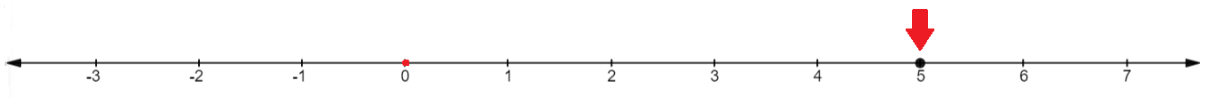(ii) $-10$

Ans: Integer is a set of both positive and negative numbers including 0.

A number line is a line on which the numbers are marked at equal intervals.

We are given an integer $-10$ to represent on the number line.

To do so, we will draw a number line and locate the given point on it.

Therefore, $-10$ represented on the number line is as follows,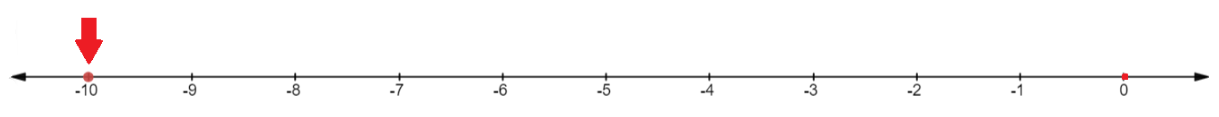(iii) $+8$

Ans: Integer is a set of both positive and negative numbers including 0.

A number line is a line on which the numbers are marked at equal intervals.

We are given an integer $+8$ to represent on the number line.

To do so, we will draw a number line and locate the given point on it.

Therefore, $+8$ represented on the number line is as follows,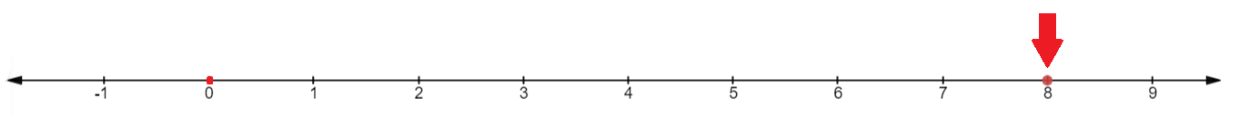(iv) $-1$

Ans: Integer is a set of both positive and negative numbers including 0.

A number line is a line on which the numbers are marked at equal intervals.

We are given an integer $-1$ to represent on the number line.

To do so, we will draw a number line and locate the given point on it.

Therefore, $-1$ represented on the number line is as follows,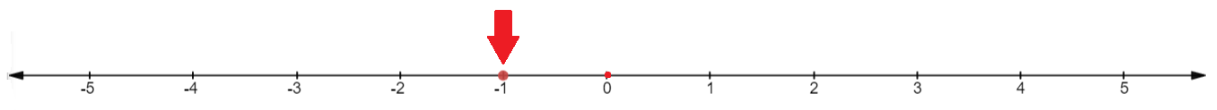(v) $-6$

Ans: Integer is a set of both positive and negative numbers including 0.

A number line is a line on which the numbers are marked at equal intervals.

We are given an integer $-6$ to represent on the number line.

To do so, we will draw a number line and locate the given point on it.

Therefore, $-6$ represented on the number line is as follows,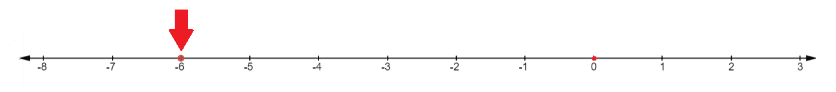4. Adjacent figure is a vertical number line, representing integers. Observe it and locate the following points: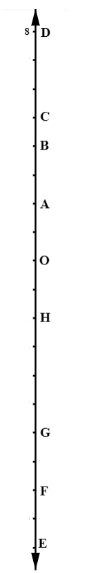(i) If point $D$ is $+8$ then which point is $-8$?

Ans: Integer is a set of both positive and negative numbers including 0.

A number line is a line on which the numbers are marked at equal intervals.

We are given a vertical number line. The numbers are arranged in a descending order on a vertical number line from top to bottom.

It is given that point $D$ represents $+8$ on the number line. It is required to tell which point represents $-8$.

To do so, mark point $D$ as $+8$. Then mark all the intervals with integers in descending order on the number line and check which point coincides with the number $-8$.

The fully labelled number line is obtained as shown below.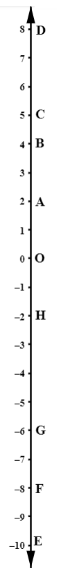From the above number line, it can be seen that $-8$ coincides with point $F$.

Therefore, point $F$ represents $-8$ on the given number line.

(ii) Is point $G$ a negative integer or a positive integer?

Ans: Integer is a set of both positive and negative numbers including 0.

A number line is a line on which the numbers are marked at equal intervals.

We are given a vertical number line. The numbers are arranged in a descending order on a vertical number line from top to bottom.

It is given that point $D$ represents $+8$ on the number line. It is required to tell which type of number point $G$ represents.

To do so, mark point $D$ as $+8$. Then mark all the intervals with integers in descending order on the number line and check which number coincides with point $G$. Then determine the type of the number, i.e., whether it is positive or negative.

The fully labelled number line is obtained as shown below.From the above number line, it can be seen that point $G$ coincides with the number $-6$. And we know that the number $-6$ is a negative number.

Therefore, point $G$ represents a negative number on the given number line.

(iii) Write integers for points $B$ and $E$.

Ans: Integer is a set of both positive and negative numbers including 0.

A number line is a line on which the numbers are marked at equal intervals.

We are given a vertical number line. The numbers are arranged in a descending order on a vertical number line from top to bottom.

It is given that point $D$ represents $+8$ on the number line. It is required to tell which integers represent points $B$ and $E$.

To do so, mark point $D$ as $+8$. Then mark all the intervals with integers in descending order on the number line and check which numbers coincide with points $B$ and $E$.

The fully labelled number line is obtained as shown below.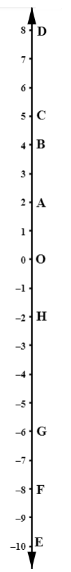From the above number line, it can be seen that point $B$ coincides with integer 4 and point $E$ coincides with integer $-10$ on the number line.

Therefore, point $B$ represents the integer 4 and point $E$ represents the integer $-10$ on the given number line.

(iv) Which point marked on this number line has the least value?

Ans: Integer is a set of both positive and negative numbers including 0.

A number line is a line on which the numbers are marked at equal intervals.

We are given a vertical number line. The numbers are arranged in a descending order on a vertical number line from top to bottom.

It is given that point $D$ represents $+8$ on the number line. It is required to tell which point on the given number line has the lowest value.

To do so, mark point $D$ as $+8$. Then mark all the intervals with integers in descending order on the number line and check which point comes at the last. This is because the number line is in descending order so the number at the end will have the least value.

The fully labelled number line is obtained as shown below.From the above number line, it can be seen that the number at the end is $-10$. The point that coincides with this number is point $E$.

Therefore, point $E$ has the lowest value on the given number line.

(v) Arrange all the points in decreasing order of values.

Ans: Integer is a set of both positive and negative numbers including 0.

A number line is a line on which the numbers are marked at equal intervals.

We are given a vertical number line. The numbers are arranged in a descending order on a vertical number line from top to bottom.

It is given that point $D$ represents $+8$ on the number line. It is required to arrange all the points in the decreasing order of values.

To do so, mark point $D$ as $+8$. Then mark all the intervals with integers in descending order on the number line and check which point coincides with which number. Arrange the points and the numbers respectively in descending order using the number line.

The fully labelled number line is obtained as shown below.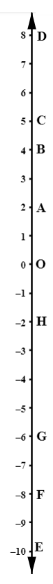From the above number line, it can be seen that the values are present in the descending order. So, we will list all the points from top to bottom to give the decreasing order of points.

Therefore, the decreasing order of point is as follows,

$D>C>B>A>O>H>G>F>E$

5. Following is the list of temperatures of five places in India, on a particular day of the year.

Place                                   Temperature

Siachen                                $10{}^\circ \text{C}$ below $0{}^\circ \text{C}$                        __________

Shimla                                 $2{}^\circ \text{C}$ below $0{}^\circ \text{C}$                          __________

Ahmedabad                       $30{}^\circ \text{C}$ above $0{}^\circ \text{C}$                        __________

Delhi                                    $20{}^\circ \text{C}$ above $0{}^\circ \text{C}$                        __________

Srinagar                               $5{}^\circ \text{C}$ below $0{}^\circ \text{C}$                          __________

(i) Write the temperature of these places in the form of integers in the blank column.

Ans: Integer is a set of both positive and negative numbers including 0.

We are given a list of five cities in India along with their temperatures. The temperatures are given w.r.t to $0{}^\circ \text{C}$. It is required to write the temperatures in the form of integers.

To do so, we will assume that all the temperatures above $0{}^\circ \text{C}$ are positive and all the temperatures below $0{}^\circ \text{C}$ are negative. Based on this concept we will fill the integers in the blanks as follows.

Siachen                                $10{}^\circ \text{C}$ below $0{}^\circ \text{C}$                        $\underline{\left( - \right)10{}^\circ \text{C}}$

Shimla                                 $2{}^\circ \text{C}$ below $0{}^\circ \text{C}$                           $\underline{\left( - \right)2{}^\circ \text{C}}$

Ahmedabad                       $30{}^\circ \text{C}$ above $0{}^\circ \text{C}$                         $\underline{\left( + \right)30{}^\circ \text{C}}$

Delhi                                    $20{}^\circ \text{C}$ above $0{}^\circ \text{C}$                         $\underline{\left( + \right)20{}^\circ \text{C}}$

Srinagar                               $5{}^\circ \text{C}$ below $0{}^\circ \text{C}$                           $\underline{\left( - \right)5{}^\circ \text{C}}$

(ii) Following is the number line representing the temperature in degree Celsius.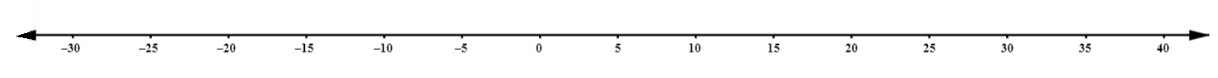Plot the name of the city against its temperature.

Ans: A number line is a line on which the numbers are marked at equal intervals.

We are given a number line that represents the temperature in degrees Celsius. It is required to plot the names of the cities against the temperatures.

To do so, we will prepare a table of cities with their temperatures.

 City Temperature Siachen $\left( - \right)10{}^\circ \text{C}$ Shimla $\left( - \right)2{}^\circ \text{C}$ Ahmedabad $\left( + \right)30{}^\circ \text{C}$ Delhi $\left( + \right)20{}^\circ \text{C}$ Srinagar $\left( - \right)5{}^\circ \text{C}$

We will now locate these temperatures on the number line and then write the names of the cities against them.

On doing so, we obtain the number line as follows,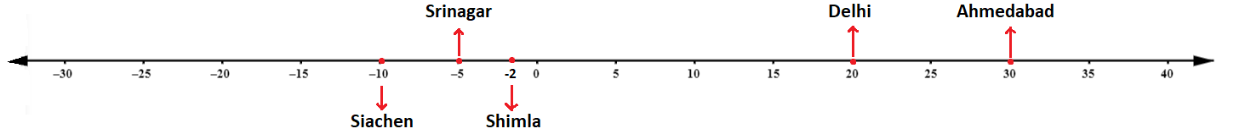(iii) Which is the coolest place?

Ans: We are given a list of five cities in India along with their temperatures. The temperatures are given w.r.t to $0{}^\circ \text{C}$. It is required to tell which is the coolest place.

To do so, we will assume that all the temperatures above $0{}^\circ \text{C}$ are positive and all the temperatures below $0{}^\circ \text{C}$ are negative. Then the number which has the lowest value will represent the coolest place.

Out of all the temperatures given, we know that $\left( - \right)10{}^\circ \text{C}$ is the lowest. Also, the place corresponding to this temperature is Siachen.

So, the coolest place is Siachen.

(iv) Write the names of the places where temperatures are above $10{}^\circ \text{C}$.

Ans: We are given a list of five cities in India along with their temperatures. The temperatures are given w.r.t to $0{}^\circ \text{C}$. It is required to tell which places have temperatures greater than $10{}^\circ \text{C}$.

To do so, we will assume that all the temperatures above $0{}^\circ \text{C}$ are positive and all the temperatures below $0{}^\circ \text{C}$ are negative. Then the numbers which are greater than $10{}^\circ \text{C}$ will have greater values of temperatures.

Out of all the temperatures given, we know that $\left( + \right)20{}^\circ \text{C}$ and $\left( + \right)30{}^\circ \text{C}$ are greater than $10{}^\circ \text{C}$. Also, the place corresponding to $\left( + \right)20{}^\circ \text{C}$ is Delhi and the place corresponding to $\left( + \right)30{}^\circ \text{C}$ is Ahmedabad.

So, the places where temperatures are above $10{}^\circ \text{C}$ are Delhi and Ahmedabad.

6. In each of the following pairs, which number is to the right of the other on the number line?

(i) 2, 9

Ans: Integer is a set of both positive and negative numbers including 0.

A number line is a line on which the numbers are marked at equal intervals.

We are given two numbers, 2 and 9. We are required to tell which numbers lie to the right of the other on the number line.

To do so, we will draw the number line and then locate both the numbers on it. We will then check which number lies to the right.

The numbers 2 and 9 located on the number line are as follows,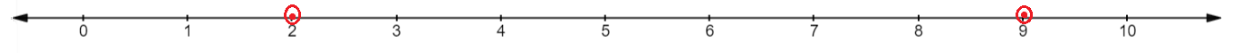From the above number line, we can see that 9 is to the right.

Therefore, 9 lies to the right of 2 on the number line.

(ii) $-3$, $-8$

Ans: Integer is a set of both positive and negative numbers including 0.

A number line is a line on which the numbers are marked at equal intervals.

We are given two numbers, $-3$ and $-8$. We are required to tell which numbers lie to the right of the other on the number line.

To do so, we will draw the number line and then locate both the numbers on it. We will then check which number lies to the right.

The numbers $-3$ and $-8$ located on the number line are as follows,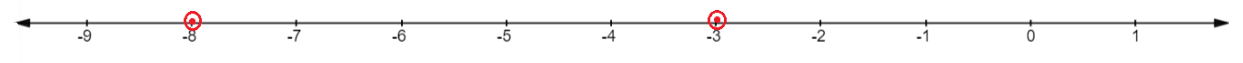From the above number line, we can see that $-3$ is to the right.

Therefore, $-3$ lies to the right of $-8$ on the number line.

(iii) 0, $-1$

Ans: Integer is a set of both positive and negative numbers including 0.

A number line is a line on which the numbers are marked at equal intervals.

We are given two numbers, 0 and $-1$. We are required to tell which numbers lie to the right of the other on the number line.

To do so, we will draw the number line and then locate both the numbers on it. We will then check which number lies to the right.

The numbers 0 and $-1$ located on the number line are as follows,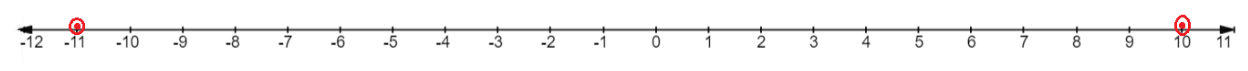From the above number line, we can see that 0 is to the right.

Therefore, 0 lies to the right of $-1$ on the number line.

(iv) $-11$, 10

Ans: Integer is a set of both positive and negative numbers including 0.

A number line is a line on which the numbers are marked at equal intervals.

We are given two numbers, $-11$ and 10. We are required to tell which numbers lie to the right of the other on the number line.

To do so, we will draw the number line and then locate both the numbers on it. We will then check which number lies to the right.

The numbers $-11$ and 10 located on the number line are as follows,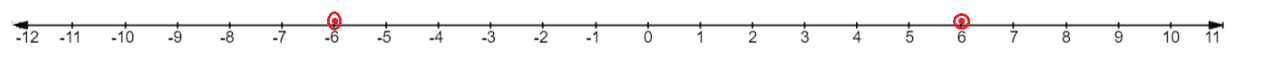From the above number line, we can see that 10 is to the right.

Therefore, 10 lies to the right of $-11$ on the number line.

(v) $-6$, 6

Ans: Integer is a set of both positive and negative numbers including 0.

A number line is a line on which the numbers are marked at equal intervals.

We are given two numbers, $-6$ and 6. We are required to tell which numbers lie to the right of the other on the number line.

To do so, we will draw the number line and then locate both the numbers on it. We will then check which number lies to the right.

The numbers $-6$ and 6 located on the number line are as follows,From the above number line, we can see that 6 is to the right.

Therefore, 6 lies to the right of $-6$ on the number line.

(vi) 1, $-100$

Ans: Integer is a set of both positive and negative numbers including 0.

A number line is a line on which the numbers are marked at equal intervals.

We are given two numbers, 1 and $-100$. We are required to tell which numbers lie to the right of the other on the number line.

To do so, we will draw the number line and then locate both the numbers on it. We will then check which number lies to the right.

The numbers 1 and $-100$ located on the number line are as follows,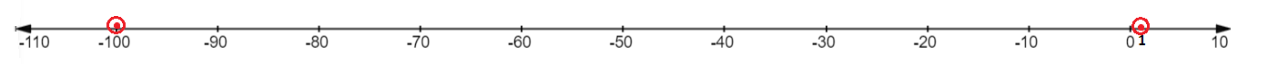From the above number line, we can see that 1 is to the right.

Therefore, 1 lies to the right of $-100$ on the number line.

7. Write all the integers between the given pairs (write them in the increasing order):

(i) 0 and $-7$

Ans: Integer is a set of both positive and negative numbers including 0.

We are required to write all the numbers in the increasing order between the pair 0 and $-7$.

We know that $-7$ is less than 0. So, we will write all the numbers from $-7$ towards 0.

The numbers in ascending order between the pair 0 and $-7$ are as follows,

$-6$, $-5$, $-4$, $-3$, $-2$, $-1$

(ii) $-4$ and 4

Ans: Integer is a set of both positive and negative numbers including 0.

We are required to write all the numbers in the increasing order between the pair $-4$ and 4.

We know that $-4$ is less than 4. So, we will write all the numbers from $-4$ towards 4.

The numbers in ascending order between the pair $-4$ and 4 are as follows,

$-3$, $-2$, $-1$, 0, 1, 2, 3

(iii) $-8$ and $-15$

Ans: Integer is a set of both positive and negative numbers including 0.

We are required to write all the numbers in the increasing order between the pair $-8$ and $-15$.

We know that $-15$ is less than $-8$. So, we will write all the numbers from $-15$ towards $-8$.

The numbers in ascending order between the pair $-8$ and $-15$ are as follows,

$-14$, $-13$, $-12$, $-11$, $-10$, $-9$

(iv) $-30$ and $-23$

Ans: Integer is a set of both positive and negative numbers including 0.

We are required to write all the numbers in the increasing order between the pair $-30$ and $-23$.

We know that $-30$ is less than $-23$. So, we will write all the numbers from $-30$ towards $-23$.

The numbers in ascending order between the pair $-30$ and $-23$ are as follows,

$-29$, $-28$, $-27$, $-26$, $-25$, $-24$

8. (i) Write four negative integers greater than $-20$.

Ans: Integer is a set of both positive and negative numbers including 0.

We are required to write four negative numbers that are greater than $-20$.

Four negative numbers that are greater than $-20$ are as follows,

$-15$, $-10$, $-5$, $-1$

(ii) Write four negative integers less than $-10$.

Ans: Integer is a set of both positive and negative numbers including 0.

We are required to write four negative numbers that are less than $-10$.

Four negative numbers that are lesser than $-10$ are as follows,

$-15$, $-20$, $-25$, $-30$

9. For the following statements write True (T) or False (F). If the statement is false, correct the statement:

(i) $-8$ is to the right of $-10$ on a number line.

Ans: Integer is a set of both positive and negative numbers including 0.

A number line is a line on which the numbers are marked at equal intervals.

We are given a statement that $-8$ is to the right of $-10$ on a number line. We are required to tell whether the given statement is true or false. If the statement is false then we are required to correct it.

To do so, we will draw the number line and then locate both the numbers on it. We will then check which number lies to the right.

The numbers $-8$ and $-10$ located on the number line are as follows,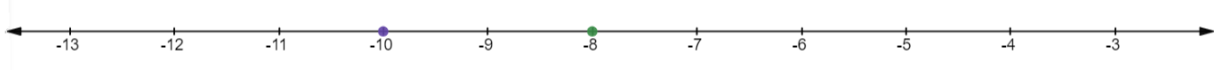From the above number line, we can see that $-8$ is to the right.

Therefore, the given statement, ‘$-8$ is to the right of $-10$ on a number line’ is true.

(ii) $-100$ is to the right of $-50$ on a number line.

Ans: Integer is a set of both positive and negative numbers including 0.

A number line is a line on which the numbers are marked at equal intervals.

We are given a statement that $-100$ is to the right of $-50$ on a number line. We are required to tell whether the given statement is true or false. If the statement is false then we are required to correct it.

To do so, we will draw the number line and then locate both the numbers on it. We will then check which number lies to the right.

The numbers $-100$ and $-50$ located on the number line are as follows,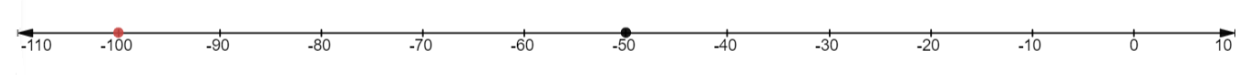From the above number line, we can see that $-50$ is to the right.

Therefore, the given statement, ‘$-100$ is to the right of $-50$ on a number line’ is false.

The corrected statement is, ‘$-50$ is to the right of $-100$ on a number line’.

(iii) Smallest negative integer is $-1$.

Ans: Integer is a set of both positive and negative numbers including 0.

We are given a statement that the smallest negative integer is $-1$. We are required to tell whether the given statement is true or false. If the statement is false then we are required to correct it.

We know that $-1$ lies to the right of all the negative numbers on the number line. And the number lying on the right is always the greatest.

Therefore, the given statement, ‘smallest negative integer is $-1$’ is false.

The corrected statement is, ‘The greatest negative integer is $-1$’.

(iv) $-26$ is larger than $-25$.

Ans: Integer is a set of both positive and negative numbers including 0.

We are given a statement that $-26$ is larger than $-25$. We are required to tell whether the given statement is true or false. If the statement is false then we are required to correct it.

We know that $-25$ lies to the right of $-26$ on the number line. And the number lying on the right is always the greatest.

So, $-25$ will be greater than $-26$.

Therefore, the given statement, ‘$-26$ is larger than $-25$’ is false.

The corrected statement is, ‘$-25$ is larger than $-26$’.

10. Draw a number line and answer the following:

(i) Which number will we reach if we move 4 numbers to the right of $-2$.

Ans: Integer is a set of both positive and negative numbers including 0.

A number line is a line on which the numbers are marked at equal intervals.

We are given a statement, ‘four numbers to the right of $-2$’. We are required to find the integer using the number line.

So, we will first locate $-2$ on the number line. We will then move four numbers to the right of $-2$.

This can be expressed on the number line as follows,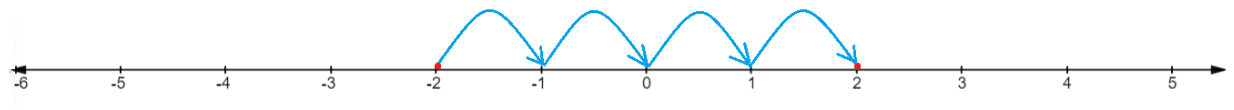Therefore, using the number line we have found that the number we reach when we move 4 numbers to the right of $-2$ is 2.

(ii) Which number will we reach if we move 5 numbers to the left of 1.

Ans: Integer is a set of both positive and negative numbers including 0.

A number line is a line on which the numbers are marked at equal intervals.

We are given a statement, ‘five numbers to the left of 1’. We are required to find the integer using the number line.

So, we will first locate 1 on the number line. We will then move five numbers to the left of 1.

This can be expressed on the number line as follows,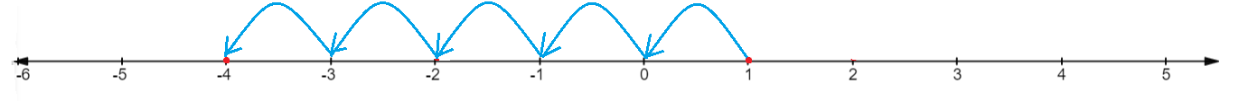Therefore, using the number line we have found that the number we reach when we move 5 numbers to the left of 1 is $-4$.

(iii) If we are at $-8$ on the number line, in which direction should we move to reach $-13$?

Ans: Integer is a set of both positive and negative numbers including 0.

A number line is a line on which the numbers are marked at equal intervals.

We are given a statement, ‘If we are at $-8$ on the number line, in which direction should we move to reach $-13$’.

So, we will first locate $-8$ on the number line. We will then move to $-13$.

This can be expressed on the number line as follows,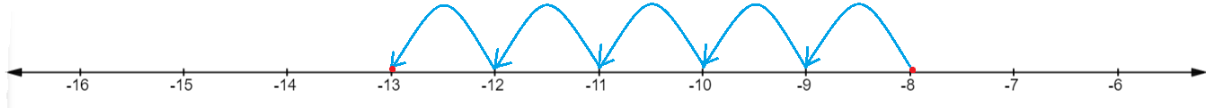Therefore, using the number line we have found that if we are at $-8$ on the number line, then we should move five numbers to the left to reach $-13$.

(iv) If we are at $-6$ on the number line, in which direction should we move to reach $-1$?

Ans: Integer is a set of both positive and negative numbers including 0.

A number line is a line on which the numbers are marked at equal intervals.

We are given a statement, ‘If we are at $-6$ on the number line, in which direction should we move to reach $-1$’.

So, we will first locate $-6$ on the number line. We will then move to $-1$.

This can be expressed on the number line as follows,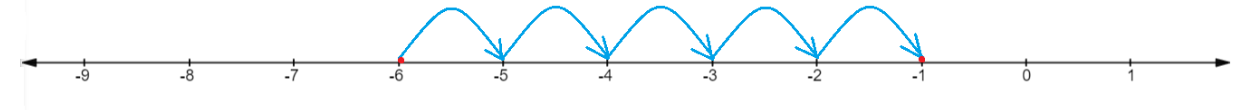Therefore, using the number line we have found that if we are at $-6$ on the number line, then we should move five numbers to the right to reach $-1$.

## NCERT Solutions for Class 6 Maths Chapter 6 Integers Exercise 6.1

Opting for the NCERT solutions for Ex 6.1 Class 6 Maths is considered as the best option for the CBSE students when it comes to exam preparation. This chapter consists of many exercises. Out of which we have provided the Exercise 6.1 Class 6 Maths NCERT solutions on this page in PDF format. You can download this solution as per your convenience or you can study it directly from our website/ app online.

Vedantu in-house subject matter experts have solved the problems/ questions from the exercise with the utmost care and by following all the guidelines by CBSE. Class 6 students who are thorough with all the concepts from the Maths textbook and quite well-versed with all the problems from the exercises given in it, then any student can easily score the highest possible marks in the final exam. With the help of this Class 6 Maths Chapter 6 Exercise 6.1 solutions, students can easily understand the pattern of questions that can be asked in the exam from this chapter and also learn the marks weightage of the chapter. So that they can prepare themselves accordingly for the final exam.

Besides these NCERT solutions for Class 6 Maths Chapter 6 Exercise 6.1, there are plenty of exercises in this chapter which contain innumerable questions as well. All these questions are solved/answered by our in-house subject experts as mentioned earlier. Hence all of these are bound to be of superior quality and anyone can refer to these during the time of exam preparation. In order to score the best possible marks in the class, it is really important to understand all the concepts of the textbooks and solve the problems from the exercises given next to it.

Do not delay any more. Download the NCERT solutions for Class 6 Maths Chapter 6 Exercise 6.1 from Vedantu website now for better exam preparation. If you have the Vedantu app in your phone, you can download the same through the app as well. The best part of these solutions is these can be accessed both online and offline as well.

1. What are integers in Maths for Class 6 Chapter 6?

As discussed in Class 6 Chapter 6, integers are the collection of whole numbers(0,1,2,3..) and the negative numbers(-1,-2,-3..). Numbers with no sign are called positive integers and numbers with a minus sign are called negative integers. Negative Integers can be used to denote temperatures, water levels in lakes, outstanding dues, etc. Integers are a new concept for Class 6 students and they should understand the basics of this chapter thoroughly as it will be useful for future classes.

2. What are the different types of integers and why are they useful?

The whole numbers and negative numbers collectively are called integers. There are two types of integers that are positive and negative. Positive numbers are written as such while negative integers are written with a minus sign in front. The concept of integers is very important because sometimes the use of a negative quantity is required, for example, the water level in lakes.

3. How do you simplify the Integers Class 6?

Integers can be a tricky concept for students of Class 6 in Chapter 6. However, it can be made easy by using the number line. The number line shows all the numbers, positive and negative, which makes it easier for students to visualize them. The number line has points at equal distances with zero at the centre. The numbers on the right side of zero are the positive integers and the numbers on the left side are the negative integers.

4. How do you learn integers easily?

Integers can be taught to students by showing them on a number line and explaining with real-life examples. As it is an advanced concept, students may find it a little difficult to understand. However, explaining with examples given in NCERT will help them in applying the concepts to real problems and they will gain confidence in this chapter. Practising the exercise questions will further strengthen their fundamentals. You can use the solutions available on the official website of Vedantu or the Vedantu App. All the resources are available free of cost.

5. How do you add and subtract integers?

The following rules are followed while adding and subtracting integers-

• On adding two positive integers, we get a positive integer

• On adding two negative integers we get a negative integer.

• When a positive and negative integer is added we subtract them as whole numbers and put the sign of the bigger number to the subtraction solution. The bigger number is the one that is big irrespective of the sign.SHARETWEETSHARESUBSCRIBE